# Lesson 3: Addition up to 10 with worksheets

Visualizing quantities using 10 frames A ten frame helps you see the answers to addition problems more easily, if you have a long string of objects like this.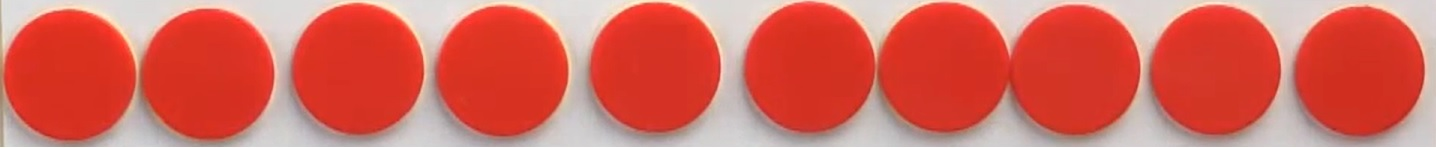Counting one by one takes too long and your eye tends to lose track of how many items you have. This is where ten frame helps.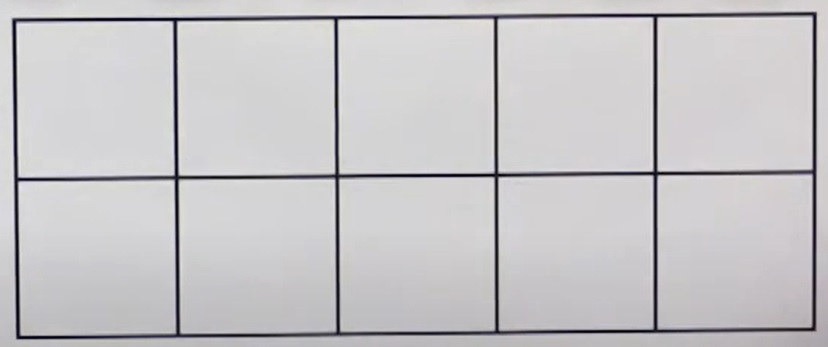A ten frame has room for ten items: five on top and five on the bottom. When you put items into a ten frame, it helps you see how many items you have at a glance without having to count one by one.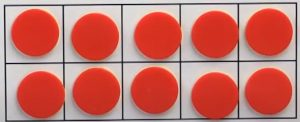So here, we can tell that we have ten counters. I take these two away, without counting one by one; we can tell that we have eight items.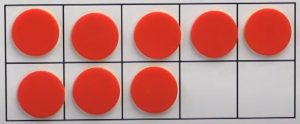We take these away, we can tell without having to count one by one that we have five items.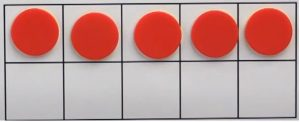So ten frame helps you see how many items you have at a glance, without having to count one by one. Part 1. Here we simply draw the correct number of dots in each ten frame. In the example, we have eight dots. Problem A asks us to draw five dots. So draw one two, three, four, five dots. Part 2. For example: here we have six, five, three, eight, two, ten, seven and 0 items. Once students are comfortable then they can write the answers. It should take them less than 45 seconds. For example: here we have six, five, three, eight, two, ten, seven and 0 items.

## Worksheets for the lessonМатематичні настільні ігри